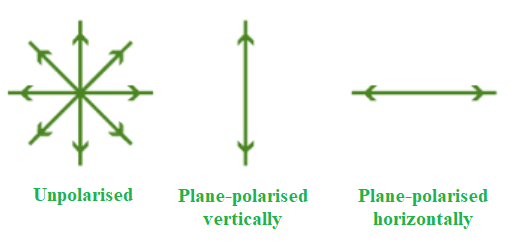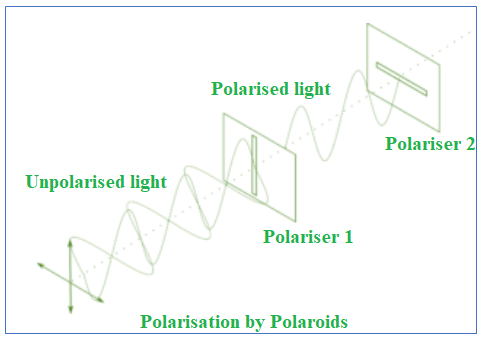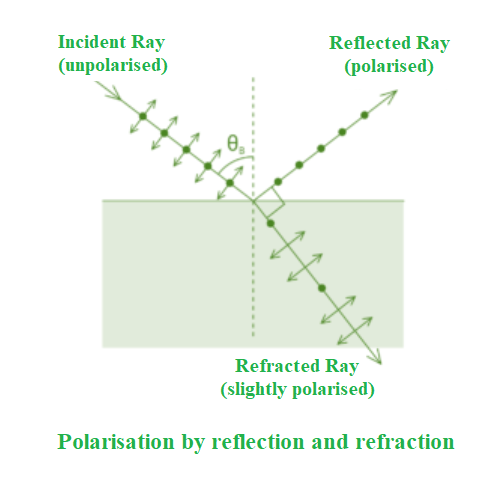Open in App
Not now

# Polarization of Light – Definition, Types, Methods, & Applications

• Difficulty Level : Basic
• Last Updated : 03 Sep, 2021

If you were to leave your house on a hot, sunny day, you would undoubtedly wear sunglasses. If you’re watching a 3D movie, you’ll also need 3D glasses. Polarisation has a significant influence in both instances. You will learn about two types of waves in this article: transverse waves and longitudinal waves. You’ll also learn about polarisation and light that is plane polarised. Let’s take a closer look at polarisation and investigate it further.

### Polarisation

Polarisation is a phenomenon induced by the wave nature of electromagnetic radiation, according to physics. Sunlight is an example of an electromagnetic wave since it travels through the vacuum to reach the Earth. Because an electric field interacts with a magnetic field, these waves are known as electromagnetic waves.

Polarisation is the process of converting non-polarized light into polarised light. The light in which particles vibrate in all various planes is known as unpolarised light.

Two types of waves are involved in this phenomenon. These are:

• Transverse waves: The waves in which the movement of the particles is perpendicular to the wave’s motion direction. For example, when you throw a stone, it creates ripples in the water and sound waves moving across the air.
• Longitudinal waves: It occurs when the medium’s particles move in the same direction as the waves.

The combination of electric and magnetic forces travelling across space is known as light. A light wave’s electric and magnetic vibrations are perpendicular to each other. The magnetic field travels in one direction and the electric field in the other, but they are always perpendicular. So we have an electric field in one plane, a magnetic field perpendicular to it, and a travel direction that is perpendicular to both. Electric and magnetic vibrations can happen in a variety of planes.

Unpolarised light is defined as a light wave that vibrates in more than one plane. Unpolarised light sources include the light emitted by the sun, a lamp, and a tube light.

A polarised wave is the opposite type of wave. Light waves that vibrate in a single plane are known as polarised waves. Plane polarised light is made up of waves with the same direction of vibration for all of them.

### Types of Polarisation

The three types of polarisation based on transverse and longitudinal wave motion are as follows:

• Linear polarisation – The electric field of light is confined to a single plane along the propagation direction in linear polarisation.
• Elliptical polarisation – The electric field of light propagates along an elliptical path. The two linear components do not have the same amplitude and phase difference.
• Circular polarisation – The electric field of light has two linear components that are perpendicular to each other and have identical amplitudes, but the phase difference is π ⁄ 2. The electric field that occurs will propagate in a circular motion.

### Methods Used in the Polarisation of Light

There are a few different ways for polarising light:

Polarisation by Polaroids

We can observe that there is a plane of vibration parallel to the plane in the diagram below. There is also a vibration plane that is perpendicular to the plane. The first picture is one that is not polarised. The second picture is polarised, meaning it is perpendicular or parallel to the first. So let’s start with polaroids to understand polarisation.Polaroids are polarising materials made up of molecules that are oriented in a specific direction. A pass axis exists on every Polaroid. Only the pass axis will enable light to flow through. Both the horizontal and vertical pass axes can exist on a polaroid. The way light passes through it is determined by these. When the light that is not polarised travels through a polaroid, it becomes polarised.Polarization by Scattering

When light strikes a molecule or an atom, the light energy is absorbed and re-emitted in multiple directions. Polarisation causes this scattering. Furthermore, the emitted light travels in many directions.

When unpolarised light is incident on a particle, we obtain dispersed light. As a result, when unpolarised light travels through a molecule, the light is polarised in the direction perpendicular to the incident ray. As a result, light polarisation occurs in this direction.

And this is how light scattering causes polarisation. The dispersed light is emitted in a direction that is perpendicular to the incident beam. Furthermore, dispersed light has complete polarisation, but light travelling through molecules has partial polarisation.

Polarisation by Reflection and Refraction

The incident ray reflected ray, and refracted ray may all be seen in the diagram below. Unpolarised light is visible on the incident beam. The unpolarized light is depicted in the diagram above. The dot denotes perpendicular directions, whereas the lines denote parallel directions.The majority of the light in the reflected ray is polarised parallel to the plane, with only a few exceptions. In contrast, most of the light in a refracted beam is unpolarized, with one or two polarised components. As a result, we can see that the reflected and refracted rays are both partly polarised.

### Brewster’s law

The law says that the reflected ray is fully polarised at a specific angle of incidence. The angle between the reflected and refracted rays is also 90°. Total angle = 90° if i = iB, that is when the angle of incidence equals Brewster’s angle.

According to Snell’s law,

μ = sin i ⁄ sin r

When light is incident at Brewster’s angle, then

iB + r  =  90°

r = 90° − iB

sin r = sin (90° − iB) = cos iB

Substitute value of sin r in the formula of μ.

μ = sin iB ⁄ cos iB

μ = tan iB

### Applications of Polarisation

The following are some examples of polarisation applications:

• In chemistry, polarisation methods are used to determine the chirality of organic molecules.
• Differentiating between transverse and longitudinal waves is done via polarisation.
• In the plastics industry, Polaroid filters are used to conduct stress analysis testing.
• Polarisation is used to create and display three-dimensional movies.
• In sunglasses, polarization is used to minimise glare.
• It’s used to analyse earthquakes in seismology.
• Polarisation is used in infrared spectroscopy.

### Sample Questions

Question 1: A beam of light strikes the surface of a plate of glass with a refractive index of √3 at the polarising angle. What will be the ray’s angle of refraction?

Consider iP be the polarising angle,

Refractive index, μ = tan iP = √3

⇒ iP = 60°

Angle of refraction, r = 90° –  iP

= 90° – 60° = 30°

Hence, the angle of refraction is 30°.

Question 2: Does the magnitude of the electric field vector vary in the case of linearly polarised light?

The magnitude of the electric field vector fluctuates regularly with time in any form of light, whether polarised or unpolarised.

Question 3: Why do the light waves exhibit polarisation but sound waves do not exhibit polarization?

Polarisation can only be seen in transverse waves. Sound waves cannot be polarised because light waves are transverse waves while sound waves are longitudinal waves.

Question 4: In a polarised wave, what is the direction in which the electric vectors oscillate?